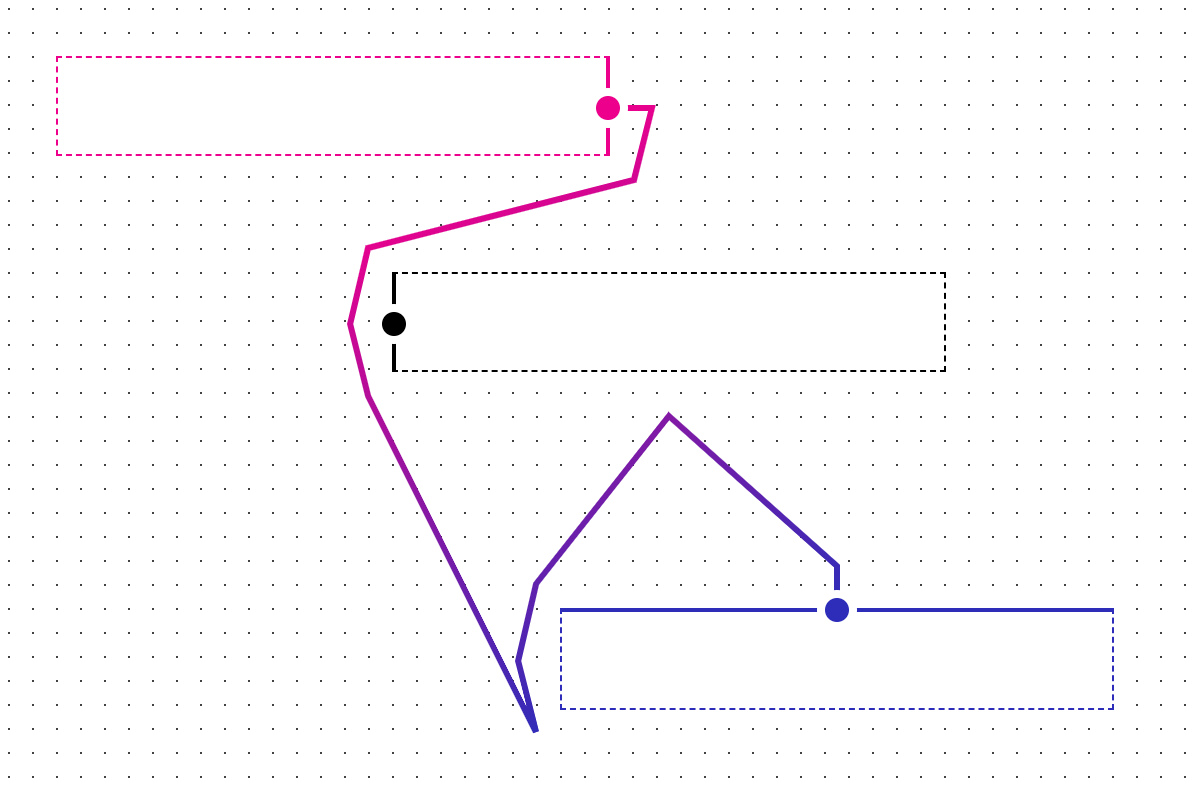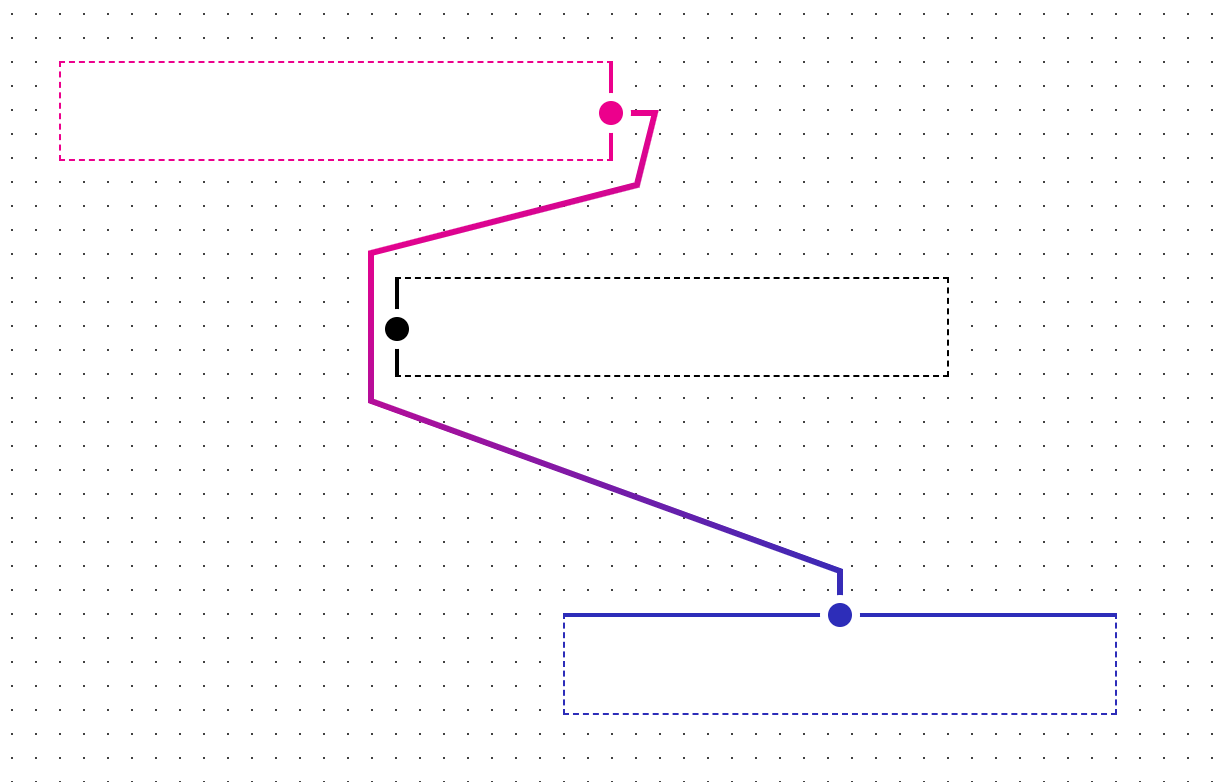## Archive for January, 2021

### A* path optimization

The A* algorithm, using a straight-line distance heuristic function, is great in terms of performance, but yields a number of cases where the paths produced are not optimal.

For example, here is a path created in ScratchGraph using GraphPaper (the underlying library used for creating the connectors):I suspect the non-optimal result is even more pronounced here given that the path is determined based on specific routing points that exist around the objects (you can spot these as the inflection points in the path), instead of a uniform grid. That said, I don’t want to use a uniform grid; while it’s likely a non-issue with A*, there is also the cost of computing which routing points are accessible vs blocked, and that cost grows quickly as the possible number of routing points grow.

An approach that works well, and doesn’t drastically increase the cost of path computation, is simplifying the generated path by checking if corresponding points in the path are visible to each other. If so, we can remove the intermediate points, and simply connect those 2 points together. Here’s a snipped from the GraphPaper codebase:

```/** * * @param {Point[]} _pointsInRoute * @param {Function} _arePointsVisibleToEachOther */ optimize: function(_pointsInRoute, _arePointsVisibleToEachOther) { let start = 0; let end = _pointsInRoute.length - 1; while(true) { if((end-start) <= 1) { start++; end = _pointsInRoute.length - 1; if(start >= _pointsInRoute.length-2) { break; } } if(_arePointsVisibleToEachOther(_pointsInRoute[start], _pointsInRoute[end])) { _pointsInRoute.splice(start + 1, (end-start) - 1); end = _pointsInRoute.length - 1; } else { end--; } } }```

The function works as follows:

• Begin with the points `start` (first point in the path) and `end` (last point in the path).
• Relative to `start`, check if the other points in the path are visible to it (in the code above, we iterate backwards from the endpoint). If we find that `start` is visible to another point, we eliminate the intermediate points from the path.
• Once we get to `end` being the point after `start`, update `start` to the next point in the path and reset `end` to whatever the last point in the path is.
• Repeat the latter 2 steps until we’ve checked all corresponding points in the path (`start` is the point directly preceding `end`).

Optimizing the path shown above with this method yields an optimal path:In terms of performance, the cost is based on the number of points in the path and the cost of whatever computation the `_arePointsVisibleToEachOther()` function does. For my use-cases, paths have relatively few points and `_arePointsVisibleToEachOther()` consists of a number of fairly fast line-line intersection checks, so the method is fairly cheap and there’s no significant decrease in performance when generating paths.